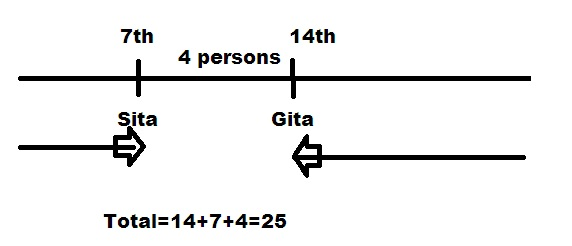# Ranking and Order Online Quiz

Following quiz provides Multiple Choice Questions (MCQs) related to Ranking and Order. You will have to read all the given answers and click over the correct answer. If you are not sure about the answer then you can check the answer using Show Answer button. You can use Next Quiz button to check new set of questions in the quiz.Q 1 - Raj and Shini are ranked 7th and 11th respectively from the top among 31 students. Determine their ranks from the bottom.

Options :

A - 23 and 20

B - 25 and 20

C - 25 and 21

D - None of these

### Explanation

Total numbers of students present behind the rank of Raj = 31 - 7 = 24

Therefore Raj’s rank is 25th from below.

Now calculating for Shini = 31 - 11 = 20

This means that Shini’s rank will be 21st from bottom.

Q 2 - Number of girls are standing in a row. Between Sita and Gita, 4 number of girls are present. From the left hand side, the rank of Sita is 14 and from right hand side, the rank of Gita is 7th. Calculate the total number of girls.

Options :

A - 23

B - 24

C - 25

D - 26

### ExplanationQ 3 - In a list, the rank of Mahesh was 16 from the top and whereas from bottom, the rank is 29. The list was about the passed-out students. Six students were failed due to non participation and 5 were failed. Find the total number of students present in the class.

Options :

A - 55

B - 54

C - 53

D - 52

### Explanation

Total number of passed out students including Mahesh is

= 29 + 16 - 1 = 44

Now adding the failed students into it = 44 + (5 + 6) = 55 is the total number of students.

Q 4 - Rank of Sujay is 14th from the top in a group of 25 students. Find his rank from below if 3 new students will be added to the bottom of the list.

Options :

A - 15

B - 11

C - 12

D - 13

### Explanation

Sujay’s current rank from below in a group of 25 students is

= (25 - 14) + 1

= 12

Now as 3 students will be added to the bottom of the list, the new rank of Sujay from bottom = 12 + 3 = 15

Q 5 - Rank of Madhaba is 14th from the bottom in a list of 64 boys. What would be his new rank from the top if 3 new boys will be added to the bottom of the list?

Options :

A - 50

B - 51

C - 52

D - 54

### Explanation

Total number of students in the row = 64

Rank of Madhaba from the bottom = 14

So, rank of Madhaba from top would be = (64 - 14) + 1 = 51

Now as adding of new boys to the bottom of the list will not affect the rank of Madhaba from top, hence; his rank will be the same 51 from the top, even after the addition.

Q 6 - Deepak’s progress report card showed that his rank is 10th among 50 students. Calculate the rank of Deepak from below.

Options :

A - 39

B - 40

C - 42

D - 41

### Explanation

The rank of Deepak can be calculated as = (50 - 10) + 1 = 41

Q 7 - The rank of X is 6th from left and rank of Y is 18th from right hand side in a row of letters. Calculate the extra number of letters required to make it a group of 30, if there are 6 letters present in between X and Y.

Options :

A - 3

B - 5

C - 7

D - None of these

### Explanation

As per ranking of Y and X and the presence of 6 letters in between them, the total number of letters in the row will be

= 6 + 18 + 6

= 30

Now we can see that it is already a group of 30 letters. Hence; no more letters are required to be added to the group.

Q 8 - Out of 50 students, rank of Sanjay is 23rd from top. What will be his rank from below?

Options :

A - 34

B - 28

C - 36

D - 37

### Explanation

This can be simply calculated as = (total students - rank from top) + 1

Q 9 - If you are standing in a queue and found out that your rank is 6th from the front, 25th from the back and your father enquired about the total number of persons standing in the queue over the phone, what will be your answer?

Options :

A - 17

B - 18

C - 19

D - None of these

### Explanation

Total number of persons in the queue

= your rank from the front + your rank from the end - 1

= 6 + 25 - 1

= 30

Q 10 - Rank of Snigdha is 15th from the top in a list of 55 girls. What would be her new rank from the bottom, if 3 new girls will be eliminated from the back of the list?

Options :

A - 37

B - 38

C - 39

D - 40